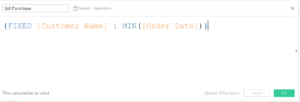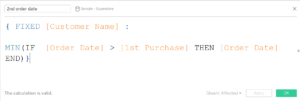by Tom Pilgrem

# Ever needed to find the 2nd value – now you can!

Sometimes we may need to find the second value in a range of dates. For example, a customers second order date. We can do this using a Level of Detail calculation (LOD).

For this example I am using superstore data – we are going to find every customers second order date. This is a useful calculation to know if we want to find out long it takes a customer to make a repeat purchase. It is worth noting that these principles could also be reversed in order to find the penultimate value as well.

## How do we do it?

###This calculation is taking the first order date at the customer level. If you are not familiar with LOD calculations, this is a fixed expression. What this means is the the calculation within the  { } is fixed at the customer level. So for each customer name, we are taking the minimum order date in the data. This gives us the first purchase date for each customer.

### 2. Calculate second orderAgain – this is a LOD calculation. If you’re not familiar with them then don’t panic! Let me walk you through it.

#### 1. Firstly – let’s take the IF statement:

IF [Order Date] > [1st Purchase] THEN [Order Date]  END

Let’s translate this into English. If the order date is greater than the first purchase, then show me the order date. Essentially what this does is exclude the first order date and ignore the very first one.

#### 2. Now Look at the Min Statement :

MIN(IF [Order Date] > [1st Purchase] THEN [Order Date]  END)

All this does is wrap up the previous IF statement in a MIN function. What this does is takes the minimum value of the previous range of dates. So instead of returning the entire range of dates excluding the first, we will see the first value in this range. So that means the second value in the data.

#### 3. The FIXED statement

{ FIXED [Customer Name] :

MIN(IF [Order Date] > [1st Purchase] THEN [Order Date]
END)}

Once again we will use a FIXED statement. What this does is say ‘For each Customer Name, perform the calculation we have just written above’. So this will return us the second value for every customer in our data.

#### Done!

That’s it. You have calculated the second value for every customer in the data. Now if you want to other calculations you can do. For example we can do a DATEDIFF calculation to calculate the difference between the two dates. Or if you want to you can change customer to product, or category, store, etc. You see where I am going with this, the possibilities are endless! And as I said at the start, we could reverse these calculations to find the penultimate value. I’m sure you can work out how!

Hope you enjoyed the post and any questions or feedback don’t hesitate to get in touch. Twitter & Linkedin.Tom Pilgrem

Thu 24 May 2018